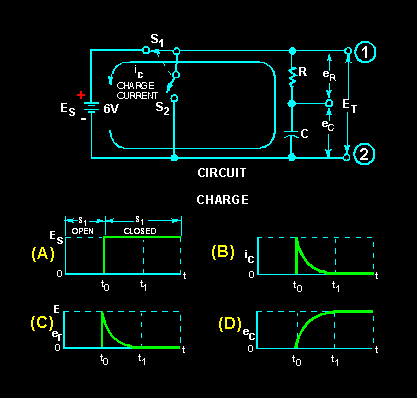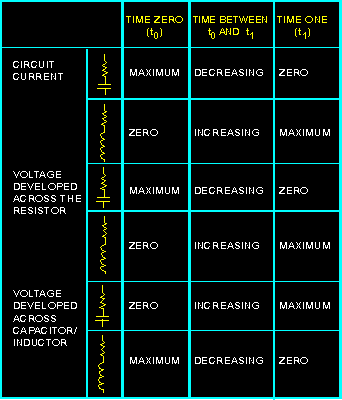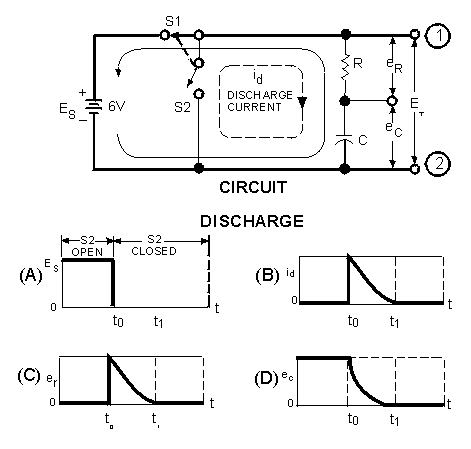Charge and discharge of an RC Series Circuit resistance is equal to the current through the resistance times the value of the resistance. This means that a voltage is developed across a resistance ONLY WHEN CURRENT FLOWS through the resistance. A capacitor is capable of storing or holding a charge of electrons. When uncharged, both plates of the capacitor contain essentially the same number of free electrons. When charged, one plate contains more free electrons than the other plate. ">Custom SearchCHARGE AND DISCHARGE OF AN RC SERIES CIRCUIT Ohm's law states that the voltage across a resistance is equal to the current through the resistance times the value of the resistance. This means that a voltage is developed across a resistance ONLY WHEN CURRENT FLOWS through the resistance. A capacitor is capable of storing or holding a charge of electrons. When uncharged, both plates of the capacitor contain essentially the same number of free electrons. When charged, one plate contains more free electrons than the other plate. The difference in the number of electrons is a measure of the charge on the capacitor. The accumulation of this charge builds up a voltage across the terminals of the capacitor, and the charge continues to increase until this voltage equals the applied voltage. The charge in a capacitor is related to the capacitance and voltage as follows:in which Q is the charge in coulombs, C the capacitance in farads, and E the emf across the capacitor in volts. CHARGE CYCLE A voltage divider containing resistance and capacitance is connected in a circuit by means of a switch, as shown at the top of figure 3-9. Such a series arrangement is called an RC series circuit. Figure 3-9. - Charge of an RC series circuit.In explaining the charge and discharge cycles of an RC series circuit, the time interval from time t0 (time zero, when the switch is first closed) to time t1 (time one, when the capacitor reaches full charge or discharge potential) will be used. (Note that switches S1 and S2 move at the same time and can never both be closed at the same time.) When switch S1 of the circuit in figure 3-9 is closed at t0, the source voltage (ES) is instantly felt across the entire circuit. Graph (A) of the figure shows an instantaneous rise at time t0 from zero to source voltage (ES = 6 volts). The total voltage can be measured across the circuit between points 1 and 2. Now look at graph (B) which represents the charging current in the capacitor (ic). At time t 0, charging current is MAXIMUM. As time elapses toward time t 1, there is a continuous decrease in current flowing into the capacitor. The decreasing flow is caused by the voltage buildup across the capacitor. At time t1, current flowing in the capacitor stops. At this time, the capacitor has reached full charge and has stored maximum energy in its electrostatic field. Graph (C) represents the voltage drop (e r) across the resistor (R). The value of er is determined by the amount of current flowing through the resistor on its way to the capacitor. At time t0 the current flowing to the capacitor is maximum. Thus, the voltage drop across the resistor is maximum (E = IR). As time progresses toward time t1, the current flowing to the capacitor steadily decreases and causes the voltage developed across the resistor (R) to steadily decrease. When time t 1 is reached, current flowing to the capacitor is stopped and the voltage developed across the resistor has decreased to zero. You should remember that capacitance opposes a change in voltage. This is shown by comparing graph (A) to graph (D). In graph (A) the voltage changed instantly from 0 volts to 6 volts across the circuit, while the voltage developed across the capacitor in graph (D) took the entire time interval from time to to time t1 to reach 6 volts. The reason for this is that in the first instant at time t 0, maximum current flows through R and the entire circuit voltage is dropped across the resistor. The voltage impressed across the capacitor at t0 is zero volts. As time progresses toward t 1, the decreasing current causes progressively less voltage to be dropped across the resistor (R), and more voltage builds up across the capacitor (C). At time t1, the voltage felt across the capacitor is equal to the source voltage (6 volts), and the voltage dropped across the resistor (R) is equal to zero. This is the complete charge cycle of the capacitor. As you may have noticed, the processes which take place in the time interval t0 to t1 in a series RC circuit are exactly opposite to those in a series LR circuit. For your comparison, the important points of the charge cycle of RC and LR circuits are summarized in table 3-1. Table 3-1. - Summary of Capacitive and Inductive Characteristics.DISCHARGE CYCLE In figure 3-10 at time t0, the capacitor is fully charged. When S1 is open and S2 closes, the capacitor discharge cycle starts. At the first instant, circuit voltage attempts to go from source potential (6 volts) to zero volts, as shown in graph (A). Remember, though, the capacitor during the charge cycle has stored energy in an electrostatic field. Figure 3-10. - Discharge of an RC Series circuit.Because S2 is closed at the same time S1 is open, the stored energy of the capacitor now has a path for current to flow. At t0, discharge current (id) from the bottom plate of the capacitor through the resistor (R) to the top plate of the capacitor (C) is maximum. As time progresses toward t1, the discharge current steadily decreases until at time t1 it reaches zero, as shown in graph (B). The discharge causes a corresponding voltage drop across the resistor as shown in graph (C). At time t0, the current through the resistor is maximum and the voltage drop (er) across the resistor is maximum. As the current through the resistor decreases, the voltage drop across the resistor decreases until at t1 it has reached a value of zero. Graph (D) shows the voltage across the capacitor (ec) during the discharge cycle. At time t 0 the voltage is maximum and as time progresses toward time t 1, the energy stored in the capacitor is depleted. At the same time the voltage across the resistor is decreasing, the voltage (e c) across the capacitor is decreasing until at time t 1 the voltage (ec) reaches zero. By comparing graph (A) with graph (D) of figure 3-10,you can see the effect that capacitance has on a change in voltage. If the circuit had not contained a capacitor, the voltage would have ceased at the instant S1 was opened at time t0. Because the capacitor is in the circuit, voltage is applied to the circuit until the capacitor has discharged completely at t1. The effect of capacitance has been to oppose this change in voltage. Q.12 At what instant does the greatest voltage appear across the resistor in a series RC circuit when the capacitor is charging? Q.13 What is the voltage drop across the resistor in an RC charging circuit when the charge on the capacitor is equal to the battery voltage?Integrated Publishing, Inc.